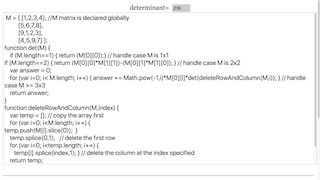### Translations

Code Language Translator Run### Software Requirements

SoftwareRequirements

 Android iOS Windows MacOS with best with Chrome Chrome Chrome Chrome support full-screen? Yes. Chrome/Opera No. Firefox/ Samsung Internet Not yet Yes Yes cannot work on some mobile browser that don't understand JavaScript such as..... cannot work on Internet Explorer 9 and below

### CreditsThis email address is being protected from spambots. You need JavaScript enabled to view it.; Francisco Esquembre; Felix J. Garcia Clemente

### Sample Learning Goals

Determinant of a matrix in JavaScript using Laplace expansion

## Determinant of 2x2 matrix

mrdaniel will be showing you how to write a function to calculate the determinant of a square matrix of any size. Let M represent the following 2x2 matrix.

var M = [ [a,b],
[c,d] ];

The following is the procedure to calculate the determinant of a 2x2 matrix.

det(M) = (a*d)-(b*c);

Now, we'll substitute the following values for the variables and write the basic det() function to calculate the determinant of a 2x2 matrix.

var M = [ [1,2],
[3,4] ];

function det(M) {
return (M*M)-(M*M);
}

// output = -2 

## Determinant of 3x3 matrix

The determinant of a 3x3 matrix becomes a bit trickier now. Let M be the following.

var M = [ [a,b,c],
[d,e,f],
[g,h,i] ];

The following is the procedure to calculate the determinant of a 3x3 matrix.

det(M) = a(ei-fh)-b(di-fg)+c(dh-eg); // notice the alternating signs

The algorithm for calculating the determinant of a 3x3 matrix is essentially the following: (1) M * determinant of the 2x2 matrix that is excluded from M's row and column. (2) M * determinant of the 2x2 matrix that is excluded from M's row and column. (3) M * determinant of the 2x2 matrix that is excluded from M's row and column. The algorithm I'm explaining is called Laplace expansion. It technically doesn't need to take the values from the first row of the matrix and multiply them by the remainder matrices-it can actually work if you use any row. This method is also very inefficient for matrices of large size. There are much more efficient ways of calculating the determinant of large square matrices. All of this is explained in the link above. So now to update our det() function to work with 3x3 matrices. We'll rewrite the function to work recursively and our base case will be if M is a 2x2 matrix, which in that case is very simple to calculate the determinant. We'll also need to write a function that deletes a specific row and column from a matrix in order to continue with the aforementioned algorithm, and for this we'll use the JavaScript splice function.

var M = [ [1,2,3],
[4,5,6],
[7,8,9] ];

function det(M) {
if (M.length==2) { return (M*M)-(M*M); }
return M*det(deleteRowAndColumn(M,0)) -
M*det(deleteRowAndColumn(M,1)) +
M*det(deleteRowAndColumn(M,2));
}

function deleteRowAndColumn(M,index) {
var temp = [];
// copy the array first
for (var i=0; i<M.length; i++) { temp.push(M[i].slice(0)); }
// delete the first row
temp.splice(0,1);
// delete the column at the index specified
for (var i=0; i<temp.length; i++) { temp[i].splice(index,1); }
return temp;
}

// output = 0

Now let's just abstract the code in det(M) into a loop rather than writing out the three separate parts. The Math.pow() function is required in order to determine the sign of the respective part since we aren't explicitly writing out minus or plus.

function det(M) {
if (M.length==2) { return (M*M)-(M*M); }
for (var i=0; i<M.length; i++) { answer += Math.pow(-1,i)*M[i]*det(deleteRowAndColumn(M,i)); }
}

## Determinant of 4x4 and larger matrices

The pattern simply continues now for larger matrices. Let M be the following matrix.

var M = [ [a,b,c,d],
[e,f,g,h],
[i,j,k,l],
[m,n,o,p] ];

The determinant of M will therefore be:

det(M) = a*det([[f,g,h],[j,k,l],[n,o,p]])-b*det([[e,g,h],[i,k,l],[m,o,p]])+...

So because our code from above runs recursively, it should actually work for a matrix of any size 4x4 or larger. Below is the final code to determine the determinant of any size square matrix.

var M = [ [1,2,3,4],
[5,6,7,8],
[9,1,2,3],
[4,5,9,7] ];

function det(M) {
if (M.length==1) { return (M);} // to cater to M case    if (M.length==2) { return (M*M)-(M*M); }
for (var i=0; i< M.length; i++) { answer += Math.pow(-1,i)*M[i]*det(deleteRowAndColumn(M,i)); }
}

function deleteRowAndColumn(M,index) {
var temp = [];
for (var i=0; i<M.length; i++) { temp.push(M[i].slice(0)); }
temp.splice(0,1);
for (var i=0; i<temp.length; i++) { temp[i].splice(index,1); }
return temp;
}

### For Teachers

The determinant of a matrix can be defined in terms of determinant of sub-matrices within the matrix using the formula

$$|A| = \sum_{j=1}^{m}(-1)^{1+j}a_{1j}|A_{1j}|$$

Where $$a_{1j}$$ is the element in first row and column j and $$A_{1j }$$ is the determinant of the sub-matrix obtained from A by removing first row and column j.

Input: Matrix A

Algorithm:

Define the determinant recursively in terms of smaller matrices with the determinant of a 1x1 matrix being itself

|A| = |A| if |A| is 1x1 matrix

$$|A| = \sum_{j=1}^{m}(-1)^{1+j}a_{1j}|A_{1j}|$$ if |A| is 2x2 matrix

$$|A| = \sum_{j=1}^{m}(-1)^{1+j}a_{1j}|A_{1j}|$$ if |A| is 3x3 and higher matrix

Research

[text]

[text]

[text]

### end faq